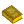## Dimension

The notion of dimension is important in mathematics because it gives a precise parameterization of the conceptual or visual complexity of any geometric object. In fact, the concept can even be applied to abstract objects which cannot be directly visualized. For example, the notion of time can be considered as one-dimensional, since it can be thought of as consisting of only now,'' before'' and after.'' Since before'' and after,'' regardless of how far back or how far into the future they are, are extensions, time is like a line, a 1-dimensional object.

To see how lower and higher dimensions relate to each other, take any geometric object (like a Point, Line, Circle, Plane, etc.), and drag'' it in an opposing direction (drag a Point to trace out a Line, a Line to trace out a box, a Circle to trace out a Cylinder, a Disk to a solid Cylinder, etc.). The result is an object which is qualitatively larger'' than the previous object, qualitative'' in the sense that, regardless of how you drag the original object, you always trace out an object of the same qualitative size.'' The Point could be made into a straight Line, a Circle, a Helix, or some other Curve, but all of these objects are qualitatively of the same dimension. The notion of dimension was invented for the purpose of measuring this qualitative'' topological property.

Making things a bit more formal, finite collections of objects (e.g., points in space) are considered 0-dimensional. Objects that are dragged'' versions of 0-dimensional objects are then called 1-dimensional. Similarly, objects which are dragged 1-dimensional objects are 2-dimensional, and so on. Dimension is formalized in mathematics as the intrinsic dimension of a Topological Space. This dimension is called the Lebesgue Covering Dimension (also known simply as the Topological Dimension). The archetypal example is Euclidean-space, which has topological dimension. The basic ideas leading up to this result (including the Dimension Invariance Theorem, Domain Invariance Theorem, and Lebesgue Covering Dimension) were developed by Poincaré,Brouwer, Lebesgue, Urysohn, and Menger.

There are several branchings and extensions of the notion of topological dimension. Implicit in the notion of the Lebesgue Covering Dimension is that dimension, in a sense, is a measure of how an object fills space. If it takes up a lot of room, it is higher dimensional, and if it takes up less room, it is lower dimensional. Hausdorff Dimension (also called Fractal Dimension) is a fine tuning of this definition that allows notions of objects with dimensions other than Integers. Fractals are objects whose Hausdorff Dimension is different from their Topological Dimension.

The concept of dimension is also used in Algebra, primarily as the dimension of a Vector Space over a Field. This usage stems from the fact that Vector Spaces over the reals were the first Vector Spaces to be studied, and for them, their topological dimension can be calculated by purely algebraic means as the Cardinality of a maximal linearly independent subset. In particular, the dimension of a Subspace ofis equal to the number of Linearly Independent Vectors needed to generate it (i.e., the number of Vectors in its Basis). Given a transformationof,See also Capacity Dimension, Codimension, Correlation Dimension, Exterior Dimension, Fractal Dimension, Hausdorff Dimension, Hausdorff-Besicovitch Dimension, Kaplan-Yorke Dimension, Krull Dimension, Lebesgue Covering Dimension, Lebesgue Dimension, Lyapunov Dimension, Poset Dimension, q-Dimension, Similarity Dimension, Topological Dimension

ReferencesDimensions

Abbott, E. A. Flatland: A Romance of Many Dimensions. New York: Dover, 1992.

Hinton, C. H. The Fourth Dimension. Pomeroy, WA: Health Research, 1993.

Manning, H. The Fourth Dimension Simply Explained. Magnolia, MA: Peter Smith, 1990.

Manning, H. Geometry of Four Dimensions. New York: Dover, 1956.

Neville, E. H. The Fourth Dimension. Cambridge, England: Cambridge University Press, 1921.

Rucker, R. von Bitter. The Fourth Dimension: A Guided Tour of the Higher Universes. Boston, MA: Houghton Mifflin, 1984.

Sommerville, D. M. Y. An Introduction to the Geometry ofDimensions. New York: Dover, 1958.